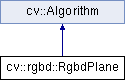OpenCV  3.1.0 Open Source Computer Vision
cv::rgbd::RgbdPlane Class Reference

#include "rgbd.hpp"

Inheritance diagram for cv::rgbd::RgbdPlane:## Public Types

enum  RGBD_PLANE_METHOD { RGBD_PLANE_METHOD_DEFAULT }

## Public Member Functions

RgbdPlane (RGBD_PLANE_METHOD method=RGBD_PLANE_METHOD_DEFAULT)

int getBlockSize () const

int getMethod () const

int getMinSize () const

double getSensorErrorA () const

double getSensorErrorB () const

double getSensorErrorC () const

double getThreshold () const

void operator() (InputArray points3d, InputArray normals, OutputArray mask, OutputArray plane_coefficients)

void operator() (InputArray points3d, OutputArray mask, OutputArray plane_coefficients)

void setBlockSize (int val)

void setMethod (int val)

void setMinSize (int val)

void setSensorErrorA (double val)

void setSensorErrorB (double val)

void setSensorErrorC (double val)

void setThreshold (double val)Public Member Functions inherited from cv::Algorithm
Algorithm ()

virtual ~Algorithm ()

virtual void clear ()
Clears the algorithm state. More...

virtual bool empty () const
Returns true if the Algorithm is empty (e.g. in the very beginning or after unsuccessful read. More...

virtual String getDefaultName () const

virtual void read (const FileNode &fn)
Reads algorithm parameters from a file storage. More...

virtual void save (const String &filename) const

virtual void write (FileStorage &fs) const
Stores algorithm parameters in a file storage. More...Static Public Member Functions inherited from cv::Algorithm
template<typename _Tp >
static Ptr< _Tp > load (const String &filename, const String &objname=String())
Loads algorithm from the file. More...

template<typename _Tp >
static Ptr< _Tp > loadFromString (const String &strModel, const String &objname=String())
Loads algorithm from a String. More...

template<typename _Tp >
static Ptr< _Tp > read (const FileNode &fn)
Reads algorithm from the file node. More...

## Detailed Description

Object that can compute planes in an image

## Constructor & Destructor Documentation

 cv::rgbd::RgbdPlane::RgbdPlane ( RGBD_PLANE_METHOD method = RGBD_PLANE_METHOD_DEFAULT )
inline

## Member Function Documentation

 int cv::rgbd::RgbdPlane::getBlockSize ( ) const
inline
 int cv::rgbd::RgbdPlane::getMethod ( ) const
inline
 int cv::rgbd::RgbdPlane::getMinSize ( ) const
inline
 double cv::rgbd::RgbdPlane::getSensorErrorA ( ) const
inline
 double cv::rgbd::RgbdPlane::getSensorErrorB ( ) const
inline
 double cv::rgbd::RgbdPlane::getSensorErrorC ( ) const
inline
 double cv::rgbd::RgbdPlane::getThreshold ( ) const
inline
 void cv::rgbd::RgbdPlane::operator() ( InputArray points3d, InputArray normals, OutputArray mask, OutputArray plane_coefficients )

Find The planes in a depth image

Parameters
 points3d the 3d points organized like the depth image: rows x cols with 3 channels normals the normals for every point in the depth image mask An image where each pixel is labeled with the plane it belongs to and 255 if it does not belong to any plane plane_coefficients the coefficients of the corresponding planes (a,b,c,d) such that ax+by+cz+d=0, norm(a,b,c)=1 and c < 0 (so that the normal points towards the camera)
 void cv::rgbd::RgbdPlane::operator() ( InputArray points3d, OutputArray mask, OutputArray plane_coefficients )

Find The planes in a depth image but without doing a normal check, which is faster but less accurate

Parameters
 points3d the 3d points organized like the depth image: rows x cols with 3 channels mask An image where each pixel is labeled with the plane it belongs to and 255 if it does not belong to any plane plane_coefficients the coefficients of the corresponding planes (a,b,c,d) such that ax+by+cz+d=0
 void cv::rgbd::RgbdPlane::setBlockSize ( int val )
inline
 void cv::rgbd::RgbdPlane::setMethod ( int val )
inline
 void cv::rgbd::RgbdPlane::setMinSize ( int val )
inline
 void cv::rgbd::RgbdPlane::setSensorErrorA ( double val )
inline
 void cv::rgbd::RgbdPlane::setSensorErrorB ( double val )
inline
 void cv::rgbd::RgbdPlane::setSensorErrorC ( double val )
inline
 void cv::rgbd::RgbdPlane::setThreshold ( double val )
inline

The documentation for this class was generated from the following file:
• /build/master-contrib_docs-mac/opencv_contrib/modules/rgbd/include/opencv2/rgbd.hpp